Question

# You throw a stone of mass m at angle θ above the horizontal with velocity v....

You throw a stone of mass m at angle θ above the horizontal with velocity v. The magnitude of the vertical acceleration of the stone the moment it leaves your hand is

g

zero

We need at least 10 more requests to produce the answer.

0 / 10 have requested this problem solution

The more requests, the faster the answer.

All students who have requested the answer will be notified once they are available.

#### Earn Coins

Coins can be redeemed for fabulous gifts.

Similar Homework Help Questions
• ### Person A throws a stone of mass m with initial velocity v, at an angle 0...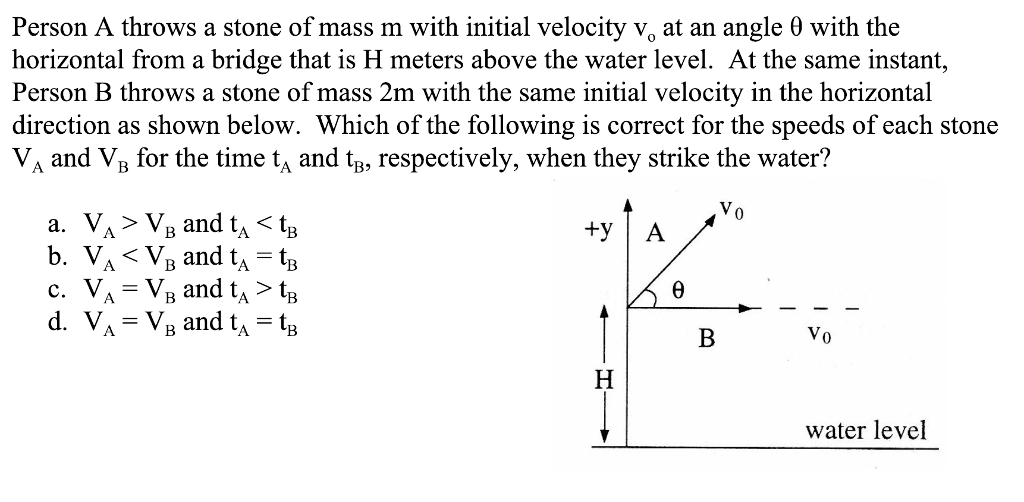Person A throws a stone of mass m with initial velocity v, at an angle 0 with the horizontal from a bridge that is H meters above the water level. At the same instant, Person B throws a stone of mass 2m with the same initial velocity in the horizontal direction as shown below. Which of the following is correct for the speeds of each stone V and Va for the time t and tn, respectively, when they strike the...

• ### A projectile is launched with an initial velocity v , at an angle θ' above the...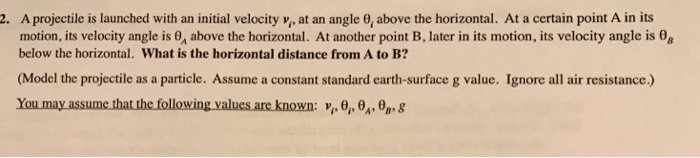A projectile is launched with an initial velocity v , at an angle θ' above the horizontal. At a certain pont A in its motion, its velocity angle is 0, above the horizontal. At another point B, later in its motion, its velocity angle is θ8 below the horizontal. What is the horizontal distance from A to B? 2. (Model the projectile as a particle. Assume a constant standard earth-surface g value. Ignore all air resistance.) You may assume that...

• ### show work round 0.0001 Question 6 (1 point) You throw a stone at a certain angle...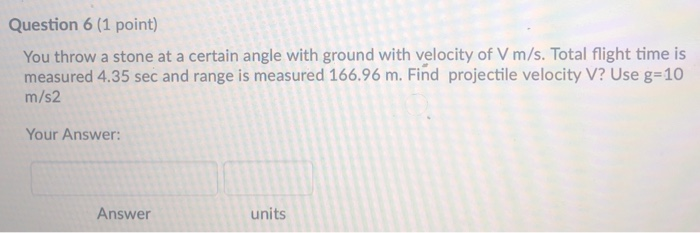show work round 0.0001 Question 6 (1 point) You throw a stone at a certain angle with ground with velocity of V m/s. Total flight time is measured 4.35 sec and range is measured 166.96 m. Find projectile velocity V? Use g=10 m/s2 Your Answer: Answer units Question 7 (0.5 points) You throw a stone vertically upward from the roof of an 31 m tall building with a velocity of 50 m/s. How long after the stone will land the...

• ### A baseball is thrown at an angle = 21° above the horizontal with an initial vertical velocity v0y = 9.75 m/s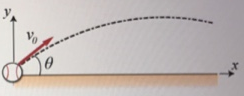A baseball is thrown at an angle = 21° above the horizontal with an initial vertical velocity v0y = 9.75 m/s. Use a Cartesian coordinate system with the origin at the baseball's initial position.Part (a) Create an expression for the ball's initial horizontal velocity component, v0x, in terms of v0y, sin(θ), and cos(θ).Part (b) Calculate the initial horizontal velocity component, v0x in m/s. Part (c) Find the ball's initial velocity magnitude, v0 in m/s.

• ### A stone thrown off a bridge 20m above a river has an initial velocity 13 m/s of at an angle of...Astone thrown offa bridge 20m above a river has an initial velocity 13 m/s of at an angle of 45^circ above the horizontal (see the figure ).1.What is the range of the stone?Express your answer using two significant figures.R=m2.At what velocity does the stone strike the water?Express your answer using two significant figures.v=m/s

• ### An object with mass m is dragged along a horizontal plane by a force acting along a rope attached to the object as shown below mass horizontal plane If the rope makes an angle θ with the plane, then...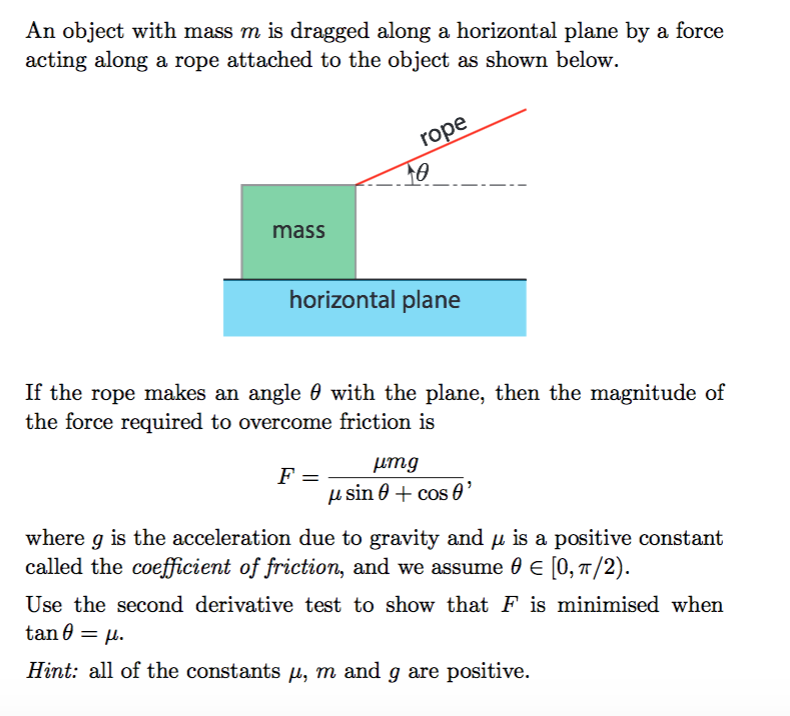An object with mass m is dragged along a horizontal plane by a force acting along a rope attached to the object as shown below mass horizontal plane If the rope makes an angle θ with the plane, then the magnitude of the force required to overcome friction is where g is the acceleration due to gravity and μ is a positive constant called the coefficient of riction, and we assume θ [0, π/2) Use the second derivative t tan...

• ### A ball with an initial velocity of 11.5 m/s and mass 1.9 kg moves at an angle 65.5° above the horizontal.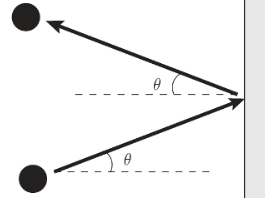A ball with an initial velocity of 11.5 m/s and mass 1.9 kg moves at an angle 65.5° above the horizontal. The ball hits a vertical wall and bounces off so that it is moving 65.5° above the horizontal, moving in the opposite direction with the same speed. See the figure. What was the magnitude of the impulse delivered by the wall, in kilogram meters per second?

• ### You throw a ball towards a wall at speed 18 m/s and at an angle 40.0...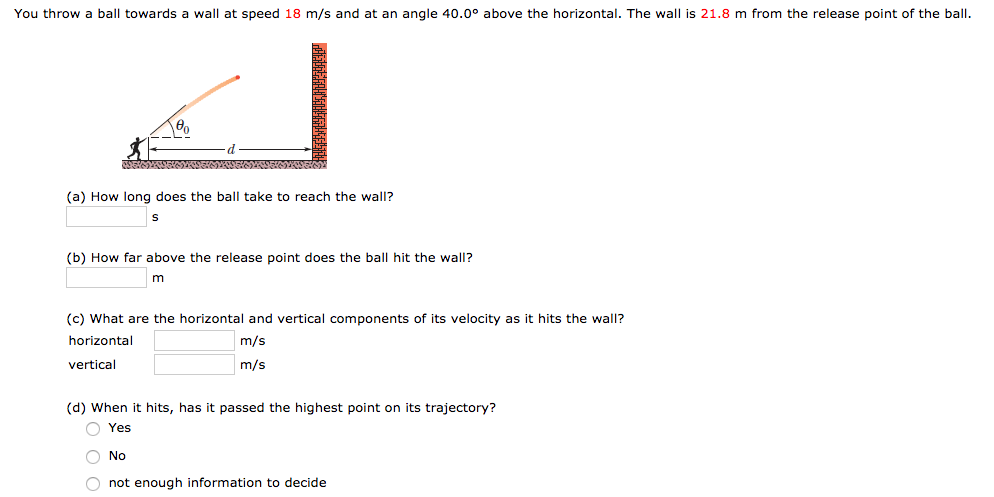You throw a ball towards a wall at speed 18 m/s and at an angle 40.0 degree above the horizontal. The wall is 21.8 m from the release point of the ball. (a) How long does the ball take to reach the wall? (b) How far above the release point does the ball hit the wall? m (c) What are the horizontal and vertical components of its velocity as it hits the wall? horizontal m/s vertical m/s (d) When it...

• ### You throw a rock with an initial horizontal velocity and it hits the ground at x...

You throw a rock with an initial horizontal velocity and it hits the ground at x meters away from your feet. What if: (a) You throw the same stone with the same initial conditions but instead you do this on a another planet with a mass 4 times the mass of the earth. With what factor will x change? (b) You throw the same stone with the same initial conditions but instead you do this on a another planet with...

• ### While standing at the edge of the roof of a building, you throw a stone upward...

While standing at the edge of the roof of a building, you throw a stone upward with an initial speed of 7.39 m/s. The stone subsequently falls to the ground, which is 16.9 m below the point where the stone leaves your hand. At what speed does the stone impact the ground? How much time is the stone in the air? Ignore air resistance and take g -9.81 m/s. (This is NOT a suggestion to carry out such an experiment!)